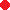Click on the points A, B, C, D to move them, avoiding to alter their counterclockwise order.

Ptolemy's theorem

(note by R. Bigoni)

A theorem of the classical plane geometry which may be a useful bridge to some of the most important theorems of the trigonometry is the so called Ptolemy's theorem:

In un quadrilateral inscribed in a circle the product of the measures of its diagonals equals the sum of the products of the measures of the opposite sides.

Demonstration (see 'cut-the-knot')

Thesis: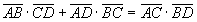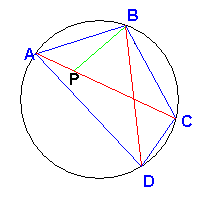Let P be the point of the diagonal AC such that the angle APB is equal to the angle DBC. The triangle APB is similar to the triangle BDC because also the angles BAP and BDC are equal since they are angles at the circumference subtended by the same arc BC.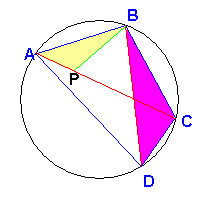The corresponding sides are in proportion, so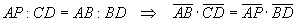Also the triangles BPC e ABD are similar because the angles PBC and ABD are equal, since they are sum of equal angles and PCB e ADB are equal since they are subtended by the same arc AB.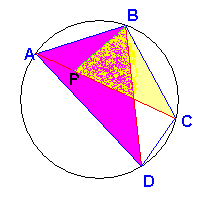The corresponding sides are in porportion therefore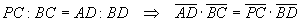The sum side by side of these two equalities gives the thesis.

From the Ptolemy's theorem we can easily deduce the Pythagoras' theorem. Indeed, every rectangle is a quadrilateral inscriptible in a circumference and its diagonals coincide with diameters.The application of the Ptolemy's theorem to the rectangle ABCD, gives immediately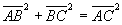that is, in the right-angled triangle ABC the sum of the squares of the catheti equals the square of the hypotenuse.

Let the quadrilateral ABCD be inscribed in a circumference and such that its diagonal AC has length 1 and coincides with a diameter.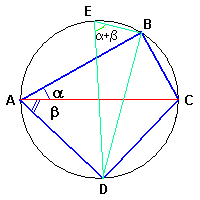The triangle ACB is right-angled, so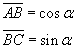The triangle ACD is right-angled, so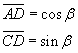If we draw the diameter DE and the chord EB, we obtain the triangle DEB which is right-angled and such that the angle DEB is equal to the angle BAD since both are angles at the circumference subtended by the same arc BD. We have therefore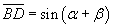If we now apply the Ptolemy's theorem to the quadrilateral ABCD we obtain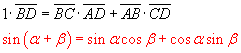This is sine addition formula from which we can deduce many other identities.

Let ABC be a triangle with sides a, b e c; let γ be the angle ACB; let we draw the circumference circumscribed to ABC and draw from a vertex, for example B, the parallel to the opposite side and let D be the point in which this parallel intersects the circumference; finally, let we draw the segment DC to obtain the quadrilateral ABDC.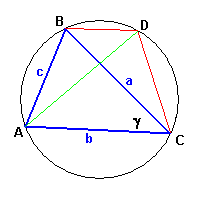The quadrilateral ABDC is an isosceles trapezoid in which the oblique sides are equal and the diagonals are equal.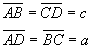Furthermore we have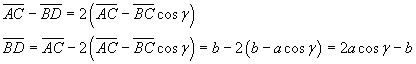The application of the Ptolemy's theorem gives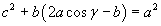and finallyThis is a very important equality, more general than the Pythagoras' theorem since it may be applied not only to right-angled triangles.

In any triangle the square of a side equals the sum of the squares of the other two sides diminished by the double of the product of the latter two sides and the cosine of the angle between them.

This equality is known as the cosine rule.

last revision: June 2015